# Limits (Evaluating)

You should read Limits (An Introduction) first

## Quick Summary of Limits

Sometimes we can't work something out directly ... but we can see what it should be as we get closer and closer!

### Example:

(x2 − 1) (x − 1)

Let's work it out for x=1:

(12 − 1) (1 − 1) = (1 − 1) (1 − 1) = 0 0

Now 0/0 is a difficulty! We don't really know the value of 0/0 (it is "indeterminate"), so we need another way of answering this.

So instead of trying to work it out for x=1 let's try approaching it closer and closer:

### Example Continued:

 x (x2 − 1) (x − 1) 0.5 1.50000 0.9 1.90000 0.99 1.99000 0.999 1.99900 0.9999 1.99990 0.99999 1.99999 ... ...

Now we see that as x gets close to 1, then (x2−1) (x−1) gets close to 2

We are now faced with an interesting situation:

• When x=1 we don't know the answer (it is indeterminate)
• But we can see that it is going to be 2

We want to give the answer "2" but can't, so instead mathematicians say exactly what is going on by using the special word "limit"

The limit of (x2−1) (x−1) as x approaches 1 is 2

And it is written in symbols as: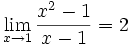So it is a special way of saying, "ignoring what happens when we get there, but as we get closer and closer the answer gets closer and closer to 2"

 As a graph it looks like this: So, in truth, we cannot say what the value at x=1 is. But we can say that as we approach 1, the limit is 2.## Evaluating Limits

"Evaluating" means to find the value of (think e-"value"-ating)

In the example above we said the limit was 2 because it looked like it was going to be. But that is not really good enough!

In fact there are many ways to get an accurate answer. Let's look at some:

### 1. Just Put The Value In

The first thing to try is just putting the value of the limit in, and see if it works (in other words substitution).

### Example: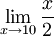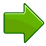102 = 5Easy!

### Example: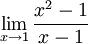(1−1)(1−1) = 00No luck.

### 2. Factors

We can try factoring.

 Example:By factoring (x2−1) into (x−1)(x+1) we get: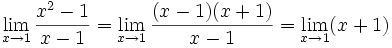Now we can just substitiute x=1 to get the limit: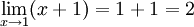### 3. Conjugate

For some fractions multiplying top and bottom by a conjugate can help.

 The conjugate is where we change the sign in the middle of 2 terms like this:Here is an example where it will help us find a limit: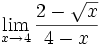Evaluating this at x=4 gives 0/0, which is not a good answer!

So, let's try some rearranging:

 Multiply top and bottom by the conjugate of the top: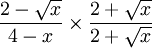Simplify top using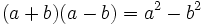: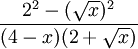Simplify top further: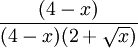Cancel (4−x) from top and bottom: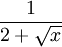So, now we have: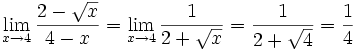Done!

### 4. Infinite Limits and Rational Functions

 A Rational Function is one that is the ratio of two polynomials: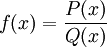For example, here P(x) = x3 + 2x − 1, and Q(x) = 6x2: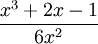By finding the overall Degree of the Function we can find out whether the function's limit is 0, Infinity, -Infinity, or easily calculated from the coefficients.

Read more at Limits To Infinity.

### 5. Formal Method

The formal method sets about proving that we can get as close as we want to the answer by making "x" close to "a".

Read more at Limits (Formal Definition)